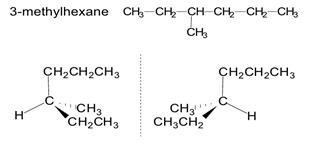Courses

# Test: Stereochemistry Level - 3

## 30 Questions MCQ Test Organic Chemistry | Test: Stereochemistry Level - 3

Description
This mock test of Test: Stereochemistry Level - 3 for Chemistry helps you for every Chemistry entrance exam. This contains 30 Multiple Choice Questions for Chemistry Test: Stereochemistry Level - 3 (mcq) to study with solutions a complete question bank. The solved questions answers in this Test: Stereochemistry Level - 3 quiz give you a good mix of easy questions and tough questions. Chemistry students definitely take this Test: Stereochemistry Level - 3 exercise for a better result in the exam. You can find other Test: Stereochemistry Level - 3 extra questions, long questions & short questions for Chemistry on EduRev as well by searching above.
QUESTION: 1

Solution:
QUESTION: 2

### Identify N and M: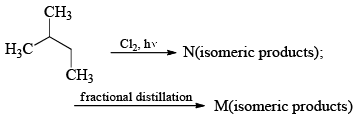Solution:

The number of isomeric products N is 6 and the number of isomeric products M is 4.  They are as shown.
M d, l cannot be separated by fractional distillation.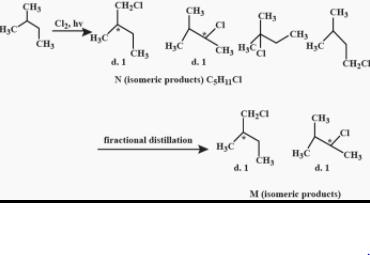QUESTION: 3

### Among the following molecules, the one that is chiral is:

Solution:

The molecules in options A, B and D contain plane of symmetry. Hence, these are achiral. The molecule in option C contains no plain of symmetry. It contains a carbon atom which is attached to four different atoms or groups. Hence, the molecule is chiral.

QUESTION: 4

The number of Diastereomers possible for the following compound is: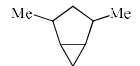Solution:

Explanation : The maximum number of diastereomers is 2n−2

n = 2

=> 2(2) - 2

= 4 - 2

= 2

QUESTION: 5

The compounds having C3-axis of symmetry are: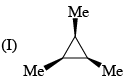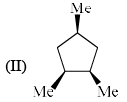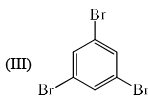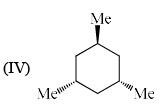Solution:
QUESTION: 6

Which one of the following conformations of cyclohexane is chiral:

Solution:
QUESTION: 7

Increasing order of stability among the three main conformations (i.e.. Eclipse, Ami, Gauche) of 2-fluoroethanol is

Solution: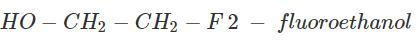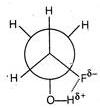Gauche conformation is comparatively more stable due to hydrogen linkage in between F and H (at O-atom), hence order is Eclipse, Anti (staggered), Gauche.

QUESTION: 8

The abso lute configuration of: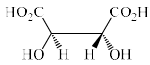Solution:
QUESTION: 9

The tautomer of II is: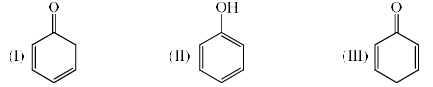Solution: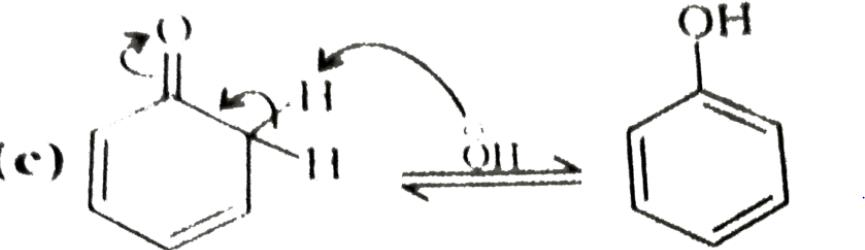(a)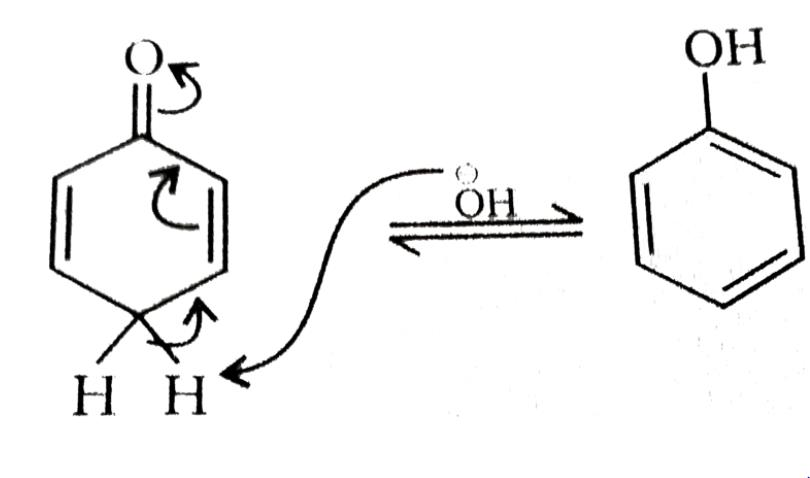QUESTION: 10

After prolonged treatment of (A) by D2O/DO, the difference in molecule weights of compounds (A) and (B) is: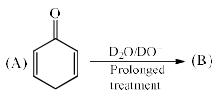Solution:

Here only the acidic hydrogen, the one attached to the double bond carbon atoms will be changed to D and the rest of the compound will remain the same.

Mass difference = 4 × 2 − 4 = 4

QUESTION: 11

The most stable conformation for the following compound is: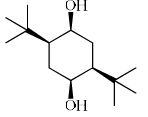Solution:
QUESTION: 12

The correct order of magnitude of ‘A value’ for the given subst ituents in cyclohexane derivatives is: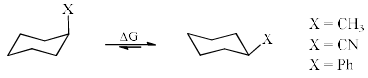Solution:
QUESTION: 13

The most stable conformation of 2-fluoroethanol is:

Solution:
QUESTION: 14

The correct statements about conformations X and Y  of 2-butanone are: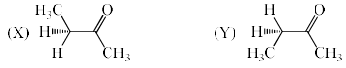(I) X is more stable than Y
(II) Y is more stable than X
(III) Methyl groups in X are anti
(IV) Methyl groups in Y are gauche

Solution:
QUESTION: 15

In the most stable conformation of neomenthol, stereochemical  orientation of the three substituents on the cyclohexane ring are:

Solution:
QUESTION: 16

The [α]D of a 90% optically pure 2-arylpropanoic acid solutions is +135°. On treatment with a base at RT for one hour, [α]D changed to +120°. The optical purity is reduced to 40% after 3 hours. If so, the optically purity of the solution after 1 hour, and its [α]D after 3 hours, respectively, would be:

Solution:
QUESTION: 17

An optically active compound enriched with R-enantiomer (60% ee) exhibited [α]D + 90°. If the, [α]D value of the sample is -135°, the ratio of R and S enantiomers would be:

Solution:
QUESTION: 18

Which of the following groups has the highest priority according to the Cahn-Ingold-Prelog sequence rules?

Solution:
*Multiple options can be correct
QUESTION: 19

The correct statement(s) concerning the structures E, F and G is (are)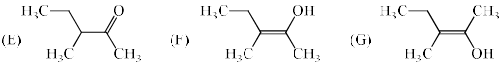Solution:
*Multiple options can be correct
QUESTION: 20

Which of the following cyclopentane derivative is/are optically active:

Solution:
*Multiple options can be correct
QUESTION: 21

Which of the following molecules is (are) chiral:

Solution:
*Multiple options can be correct
QUESTION: 22

The binaphthol (Bnp) is: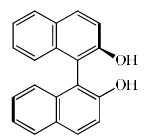Solution:
*Multiple options can be correct
QUESTION: 23

Identify which of the structures below are meso structures:

Solution:
QUESTION: 24

Among A-D, the compounds which can't exhibit optical activity are:

Solution:

From above options:

B) is meso compound, so, it won't show optical activity

A) N is sp3 hybridized to 4 different atoms connected, so, it will show optical activity

C) Sulfur is attached to 4 different atoms including lone pair and there is no chance of flipping due to large size to sulfur as compared to nitrogen, so, it will also show optical activity

D) is asymmetric carbon with for different atoms and obviously, it will show optical activity.

*Multiple options can be correct
QUESTION: 25

Consider the following statements:

Solution:
*Multiple options can be correct
QUESTION: 26

The molecule(s) that exist as meso structure(s) is/are:

Solution:

Explanation : A meso compound is a molecule that has chiral centers but also has an internal plane of symmetry. This renders the molecule achiral: it does not have an enantiomer, and it does not rotate plane polarized light . Therefore option a amd b are correct.

*Multiple options can be correct
QUESTION: 27

Amongst the following amino acids, the (S)–enantiomer is represented by:

Solution:

When the highest priority substituent to the lowest priority substituent are arranged in a clockwise manner the then the stereocenter is labeled R ("Rectus"  Latin= "right"). In an amino acid the highest priority group is NH2​ then COOH and then CH3​. In option C the amino acid has right handed configuration of substituents and therefore is R− enantiomer.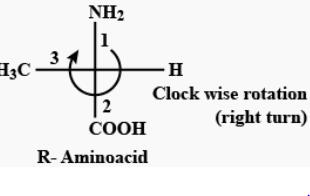QUESTION: 28

Compounds which have different arrangements of atoms in space while having the same atoms bonded to each other are said to have

Solution:

Ans: d

Explanation: Stereoisomer contrasts with structural isomers, which share the same molecular formula, but the bond connections or their order differs. By definition, molecules that are stereoisomers of each other represent the same structural isomer.

*Multiple options can be correct
QUESTION: 29

Which of the following structure is meso–2, 3–butanediol:

Solution:
QUESTION: 30

Which of the following is an alkane which can exhibit optical activity?

Solution:

3-Methylhexane shows optical activity as shown below: